# Algorithm for Generating the Flower of Life Pattern



## Section 1. Introduction and Basic Geometry.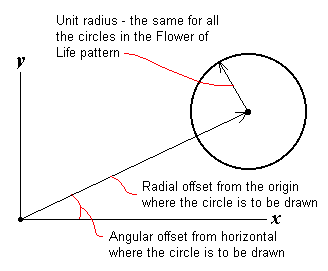The algorithms shown below describe how to calculate the origin of each unit circle for any arbitrary number of rotations. A rotation may be defined as traveling once around the perimeter of the pattern, drawing a new unit circle at the outer most point where two or more circles intersect. This process may be viewed, by analogy, as the hand of a clock sweeping around in a circle. At equally spaced times a unit circle is drawn, centered at the end of the clock hand. Thus the location each unit circle in a rotation may be determined by calculating the polar coordinates of the unit circle's center.

Since the html canvas primitive functions use rectangular coordinates, we translate the polar coordinates to rectangular coordinates using the equations

xc = radial distance  ·  cos(angular offset)
yc = radial distance  ·  sin(angular offset)
Using polar coordinates to specify the origin of each unit circle allows for the use of very simple algorithms to generate each successive rotation. The center of each unit circle is computed by calculating its radial offset from the center of the first circle and calculating its angular offset from the horizontal x axis.

In section 2, we will generalize on the algorithm described in this section. The generalized algorithm will allow drawing patterns for any arbitrary number of rotations.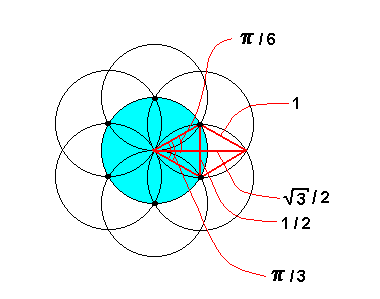### Figure 1.1 - 1st Rotation

1. φ ← π/2
2. r ← 1
3. draw unit circle centered at (r, φ)
4. φ ← φ + π/3
5. repeat steps 3 and 4 a total of five more times

Figure 1.1 shows the foundational geometry of Flower of Life patterns. Arbitrarily define the radius of each circle as 1, that is, as a unit circle. The overlap of any two circles defines two equilateral triangles each with side of length 1. Hence the angular distance between each exterior unit circle is π/3, and the distance to the outer intersect point is √3/2.

## Section 2. A Generalized Algorithm.

While section 1 of this article concentrated on a specific algorithm for the first rotation, we will now move toward generalizing this algorithm. We will define as intersects all the outermost points (with respect to the center of the first circle) where two or more circles intersect. These are all the points, where two or more circles intersect, that are most distant from the center of the first circle. We will define radius rm and r to be the distance from the center of the first circle to these intersect points. A unit circle is defined as a circle with the same radius as the first circle, that is, a radius equal to 1. All subsequent circles are drawn with this radius. The locations of unit circles are computed in polar coordinates with the center of the first circle taken as the origin. First we will look at odd numbered rotations, followed by even numbered rotations.

### 2.1 Odd Numbered Rotations

First note that all odd numbered rotations follow the same pattern. Six circles are always drawn at the same angular coordinates as in the previous, odd rotation. Only the radius r, the distance from the center of the first circle, changes for each rotation. Looking at figure 2.1, each pair of adjacent, red colored line segments defines an equilateral triangle. Hence, rotating around the pattern, each intersect point occurs at a π/3 angular increment from the previous intersect point. By induction, each intersect point is located a distance r = (n + 1)/2 from the origin, where n is the number of the rotation. With this information we have enough to define the following algorithm.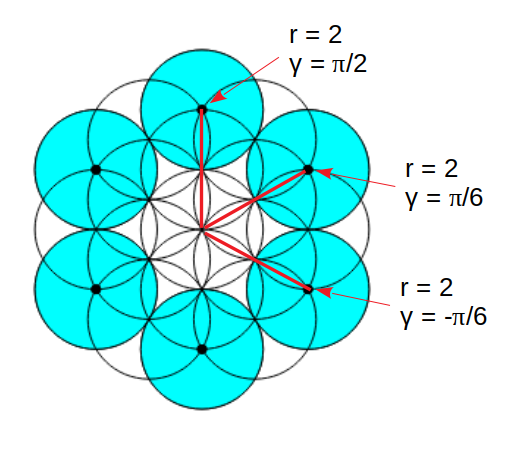Figure 2.1 - 3rd rotation
1. γ ← π/2
2. r ← (n + 1)/2
3. draw unit circle centered at (r, γ)
4. γ ← γ + π/3
5. repeat steps 3 and 4 a total of five more times

### 2.2 Even Numbered Rotations

We divide even numbered rotations into two cases. First, we consider even numbered rotations that have an even number of intersects in any given sector. A sector is defined as a sequence of intersects, a unit distance apart, that are mutually co-linear with each other. Second, we consider even numbered rotations that have an odd number of intersects in any given sector.

#### Case 1. An Even Number of Intersects.

Looking at figure 2.2, note that the number of intersects per sector equals n/2, where n is the rotation number. By symmetry, we need only consider the intersects above line segment b. The line segments b, hm, and rm form a right triangle. By induction, the line segment b has a length of √3·(n/4 + ½), and the height hm equals (m + ½), where m equals 0, … , n/4 - 1. Therefore, the angle φm equals arctan[hm  / b], and by the Pythagorean Theorem, the radius rm equals (b2 + hm 2)1/2. With this information we have enough to define the following algorithm.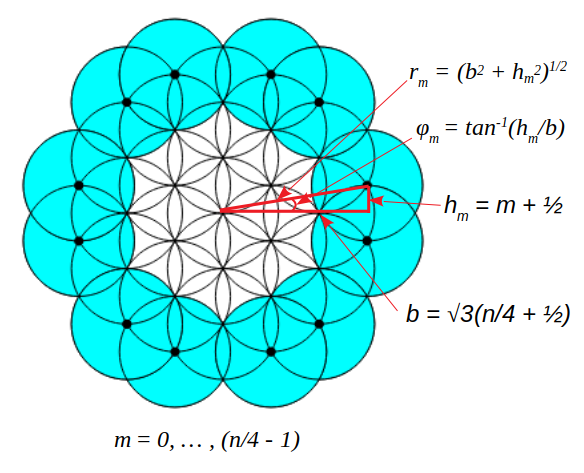Figure 2.2 - 4th rotation
1. γ ← 0
2. b ← √3·(n/4 + ½)
3. m ← 0
4. if m ≥ n/4 go to step 12
5. hm ← (m + ½)
6. φm ← tan-1[hm / b]
7. rm ← (b2 + hm 2)1/2
8. draw unit circle centered at (rm, γ + φ m)
9. draw unit circle centered at (rm, γ - φ m)
10. m ← m + 1
11. go to step 4
12. γ ← γ + π/3
13. repeat steps 3 through 12 a total of five more times

### Case 2. An Odd Number of Intersects.

Looking at figure 2.3, note once again that the number of intersects per sector equals n/2, where n is the number of the rotation. By symmetry, we need only consider the single intersect at the end of line segment b, as well as the intersects above b. The line segments b, hm, and rm form a right triangle. By induction, the line segment b has a length of √3·((n - 2)/4 + 1), and the height hm equals m, where m equals 0, … , (n - 2)/4. Therefore, the angle φm equals arctan[hm / b], and by the Pythagorean Theorem, the radius rm equals (b2 + hm2)1/2. With this information we have enough to define the following algorithm.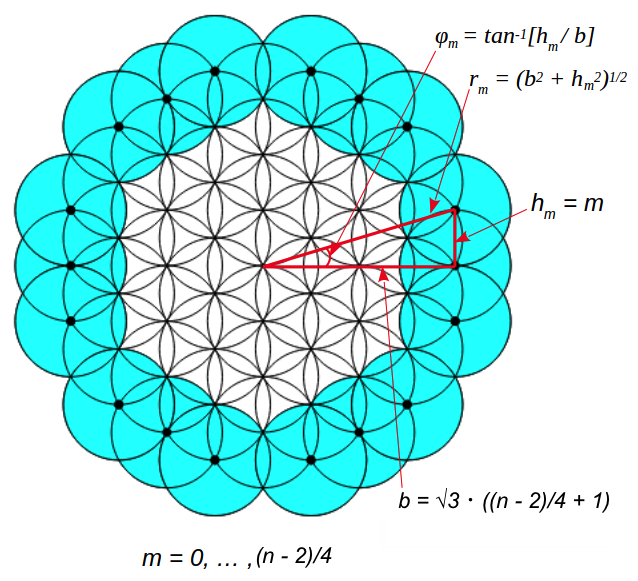Figure 2.3 - 6th rotation
1. γ ← 0
2. b ← √3·((n - 2)/4 + 1)
3. draw unit circle centered at (b, γ)
4. m ← 1
5. if m > (n - 2)/4 go to step 13
6. hm ← m
7. φm ← tan-1[hm / b]
8. rm ← (b2 + hm2 )1/2
9. draw unit circle centered at (rm, γ + φm)
10. draw unit circle centered at (rm, γ - φm)
11. m ← m + 1
12. go to step 5
13. γ ← γ + π/3
14. repeat steps 3 through 13 a total of five more times